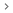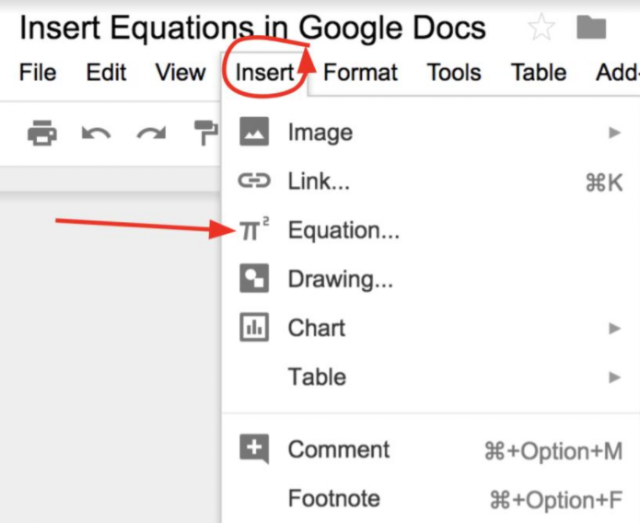# How To Make An Exponent In Google Docs

To make an equation in google docs we have to follow these simple steps which are as follows;

Insert equation

1. Open the document in Google Docs.
2. Click where you want to insert the equation.
3. Click InsertEquation.
4. Select the symbol you want to add from the corresponding menu.
5. Add numbers or substitute variables in the text box.To show or hide the equation options, click View toshow the equation toolbar.

Use Equation Shortcuts

Just enter  in the equation and add the symbol name and the space bar, you can insert the symbol. For example, type alpha to insert 𝞪.

To enter superscript or subscript, enter  and then press Shift + 6 or Shift + – .

New Equation

Therefore we will only have to enter the normal characters from the keyboard, and the special ones through these lists. Furthermore, if we are going to introduce several of these equations, when we go to the next position,

Click on the New equation button that is located to the left of these symbols.This is how easy to use exponents in google docs. I hope this brief article proved to be informative for you.

See More: